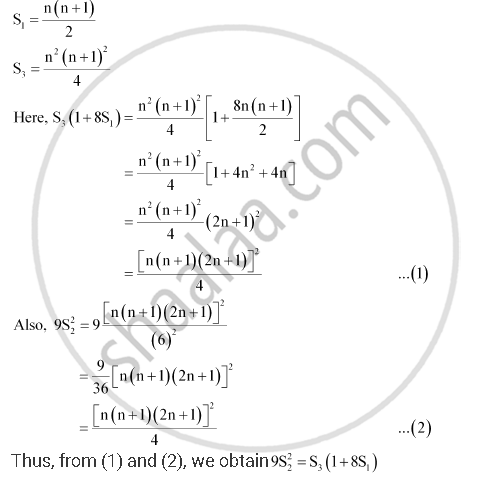CBSE (Arts) Class 11CBSE
Share
Notifications

View all notifications
Books Shortlist
Your shortlist is empty

# If S1, S2, S3 Are the Sum of First N Natural Numbers, Their Squares and Their Cubes, Respectively, Show That 9s_2^2 = S_3(1 + 8s_1) - CBSE (Arts) Class 11 - Mathematics

Login
Create free account

Forgot password?
ConceptConcept of Sequences

#### Question

If S1, S2, S3 are the sum of first n natural numbers, their squares and their cubes, respectively, show that  9S_2^2 = S_3(1 + 8S_1)

#### Solution

From the given information,Is there an error in this question or solution?

#### APPEARS IN

NCERT Solution for Mathematics Textbook for Class 11 (2018 to Current)
Chapter 9: Sequences and Series
Q: 24 | Page no. 200
Solution If S1, S2, S3 Are the Sum of First N Natural Numbers, Their Squares and Their Cubes, Respectively, Show That 9s_2^2 = S_3(1 + 8s_1) Concept: Concept of Sequences.
S